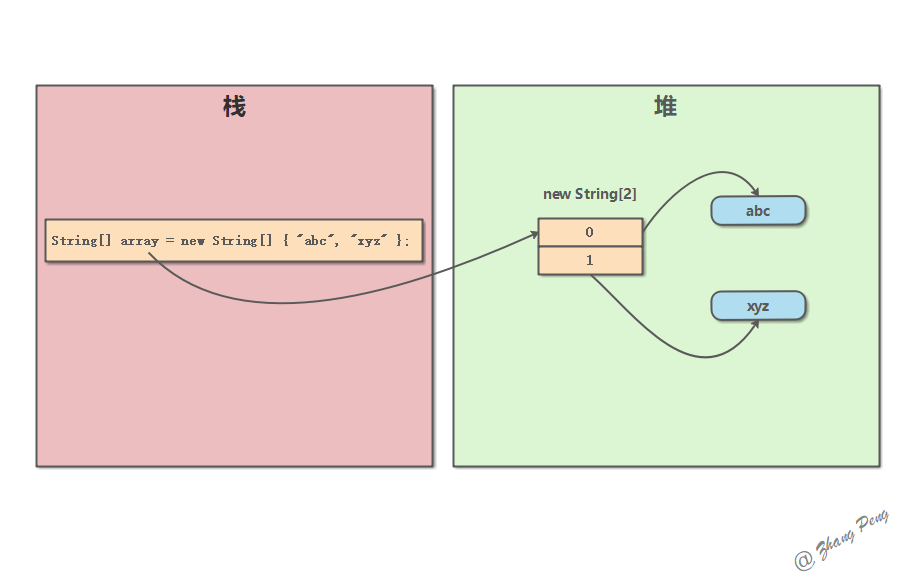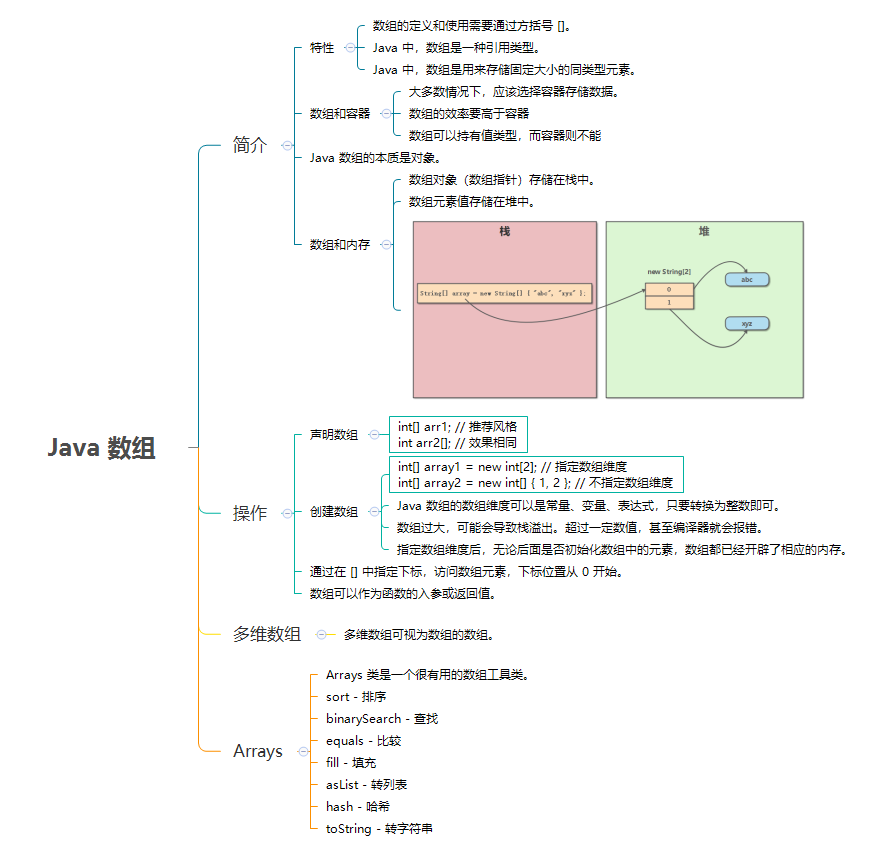# # 深入理解 Java 数组

📦 本文以及示例源码已归档在 javacore (opens new window)

## # 1. 简介

### # 1.1. 数组的特性

Java 中，数组是一种引用类型。

Java 中，数组是用来存储固定大小的同类型元素。

### # 1.2. 数组和容器

Java 中，既然有了强大的容器，是不是就不需要数组了？

• Java 中，数组是一种效率最高的存储和随机访问对象引用序列的方式。数组的效率要高于容器（如 `ArrayList`）。
• 数组可以持有值类型，而容器则不能（这时，就必须用到包装类）。

### # 1.3. Java 数组的本质是对象

Java 数组的本质是对象。它具有 Java 中其他对象的一些基本特点：封装了一些数据，可以访问属性，也可以调用方法。所以，数组是对象。

### # 1.4. Java 数组和内存

Java 数组在内存中的存储是这样的：## # 2. 声明数组

``````int[] arr1; // 推荐风格
int arr2[]; // 效果相同
``````

## # 3. 创建数组

Java 语言使用 `new` 操作符来创建数组。有两种创建数组方式：

• 指定数组维度
• 为数组开辟指定大小的数组维度。
• 如果数组元素是基础数据类型，会将每个元素设为默认值；如果是引用类型，元素值为 `null`
• 不指定数组维度
• 用花括号中的实际元素初始化数组，数组大小与元素数相同。

``````public class ArrayDemo {
public static void main(String[] args) {
int[] array1 = new int; // 指定数组维度
int[] array2 = new int[] { 1, 2 }; // 不指定数组维度

System.out.println("array1 size is " + array1.length);
for (int item : array1) {
System.out.println(item);
}

System.out.println("array2 size is " + array1.length);
for (int item : array2) {
System.out.println(item);
}
}
}
// Output:
// array1 size is 2
// 0
// 0
// array2 size is 2
// 1
// 2
``````

💡 说明 请注意数组 array1 中的元素虽然没有初始化，但是 length 和指定的数组维度是一样的。这表明指定数组维度后，无论后面是否初始化数组中的元素，数组都已经开辟了相应的内存

``````public class ArrayDemo2 {
static class User {}

public static void main(String[] args) {
User[] array1 = new User; // 指定数组维度
User[] array2 = new User[] {new User(), new User()}; // 不指定数组维度

System.out.println("array1: ");
for (User item : array1) {
System.out.println(item);
}

System.out.println("array2: ");
for (User item : array2) {
System.out.println(item);
}
}
}
// Output:
// array1:
// null
// null
// array2:
// io.github.dunwu.javacore.array.ArrayDemo2\$User@4141d797
// io.github.dunwu.javacore.array.ArrayDemo2\$User@68f7aae2
``````

💡 说明

### # 3.1. 数组维度的形式

• 数组维度可以是整数、字符。
• 数组维度可以是整数型、字符型变量。
• 数组维度可以是计算结果为整数或字符的表达式。

``````public class ArrayDemo3 {
public static void main(String[] args) {
int length = 3;
// 放开被注掉的代码，编译器会报错
// int[] array = new int[4.0];
// int[] array2 = new int["test"];
int[] array3 = new int['a'];
int[] array4 = new int[length];
int[] array5 = new int[length + 2];
int[] array6 = new int['a' + 2];
// int[] array7 = new int[length + 2.1];
System.out.println("array3.length = [" + array3.length + "]");
System.out.println("array4.length = [" + array4.length + "]");
System.out.println("array5.length = [" + array5.length + "]");
System.out.println("array6.length = [" + array6.length + "]");
}
}
// Output:
// array3.length = 
// array4.length = 
// array5.length = 
// array6.length = 
``````

💡 说明

### # 3.2. 数组维度的大小

``````int[] array = new int; // 数组维度过大，编译报错
``````

## # 4. 访问数组

Java 中，可以通过在 `[]` 中指定下标，访问数组元素，下标位置从 0 开始。

``````public class ArrayDemo4 {
public static void main(String[] args) {
int[] array = {1, 2, 3};
for (int i = 0; i < array.length; i++) {
array[i]++;
System.out.println(String.format("array[%d] = %d", i, array[i]));
}
}
}
// Output:
// array = 2
// array = 3
// array = 4
``````

💡 说明

## # 5. 数组的引用

Java 中，数组类型是一种引用类型

``````public class ArrayRefDemo {
private static void fun(int[] array) {
for (int i : array) {
System.out.print(i + "\t");
}
}

public static void main(String[] args) {
int[] array = new int[] {1, 3, 5};
fun(array);
}
}
// Output:
// 1	3	5
``````

``````public class ArrayRefDemo2 {
/**
* 返回一个数组
*/
private static int[] fun() {
return new int[] {1, 3, 5};
}

public static void main(String[] args) {
int[] array = fun();
System.out.println(Arrays.toString(array));
}
}
// Output:
// [1, 3, 5]
``````

## # 6. 泛型和数组

``````Peel<Banana>[] peels = new Pell<Banana>; // 这行代码非法
``````

Java 中不允许直接创建泛型数组。但是，可以通过创建一个类型擦除的数组，然后转型的方式来创建泛型数组。

``````public class GenericArrayDemo<T> {

static class GenericArray<T> {
private T[] array;

public GenericArray(int num) {
array = (T[]) new Object[num];
}

public void put(int index, T item) {
array[index] = item;
}

public T get(int index) { return array[index]; }

public T[] array() { return array; }
}

public static void main(String[] args) {
GenericArray<Integer> genericArray = new GenericArray<Integer>(4);
genericArray.put(0, 0);
genericArray.put(1, 1);
Object[] array = genericArray.array();
System.out.println(Arrays.deepToString(array));
}
}
// Output:
// [0, 1, null, null]
``````

## # 7. 多维数组

Java 可以支持二维数组、三维数组、四维数组、五维数组。。。

``````public class MultiArrayDemo {
public static void main(String[] args) {
Integer[][] a1 = { // 自动装箱
{1, 2, 3,},
{4, 5, 6,},
};
Double[][][] a2 = { // 自动装箱
{ {1.1, 2.2}, {3.3, 4.4} },
{ {5.5, 6.6}, {7.7, 8.8} },
{ {9.9, 1.2}, {2.3, 3.4} },
};
String[][] a3 = {
{"The", "Quick", "Sly", "Fox"},
{"Jumped", "Over"},
{"The", "Lazy", "Brown", "Dog", "and", "friend"},
};
System.out.println("a1: " + Arrays.deepToString(a1));
System.out.println("a2: " + Arrays.deepToString(a2));
System.out.println("a3: " + Arrays.deepToString(a3));
}
}
// Output:
// a1: [[1, 2, 3], [4, 5, 6]]
// a2: [[[1.1, 2.2], [3.3, 4.4]], [[5.5, 6.6], [7.7, 8.8]], [[9.9, 1.2], [2.3, 3.4]]]
// a3: [[The, Quick, Sly, Fox], [Jumped, Over], [The, Lazy, Brown, Dog, and, friend]]
``````

## # 8. Arrays 类

Java 中，提供了一个很有用的数组工具类：Arrays。

• `sort` - 排序
• `binarySearch` - 查找
• `equals` - 比较
• `fill` - 填充
• `asList` - 转列表
• `hash` - 哈希
• `toString` - 转字符串

## # 9. 小结